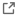# A Method of Q-Matrix Validation for the Linear Logistic Test Model

September, 2017

Article Type: Research Article

Journal Name:

DOI: 10.3389/fpsyg.2017.00897Issue: | Volume: 8 | Page No:

## Abstract

The linear logistic test model (LLTM) is a well-recognized psychometric model for examining the components of difficulty in cognitive tests and validating construct theories. The plausibility of the construct model, summarized in a matrix of weights, known as the Q-matrix or weight matrix, is tested by (1) comparing the fit of LLTM with the fit of the Rasch model (RM) using the likelihood ratio (LR) test and (2) by examining the correlation between the Rasch model item parameters and LLTM reconstructed item parameters. The problem with the LR test is that it is almost always significant and, consequently, LLTM is rejected. The drawback of examining the correlation coefficient is that there is no cut-off value or lower bound for the magnitude of the correlation coefficient. In this article we suggest a simulationmethod to set aminimum benchmark for the correlation between itemparameters fromthe Raschmodel and those reconstructed by the LLTM. If the cognitive model is valid then the correlation coefficient between the RM-based item parameters and the LLTM-reconstructed item parameters derived from the theoretical weight matrix should be greater than those derived from the simulated matrices.